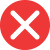3rd

Correct spelling, explanation: this form is the correct one because 3rd is a numeral form from the word third. As you can see third ends with rd and so should (and does) the numeral version of this ordinal number. The same rule applies to all other ordinal numbers, for example first is 1st and twenty-fifth is 25th. Therefore 3rd is the correct form, not 3th. The mistake probably comes from the fact that th, an ordinal number suffix, is the most common one.

Definition of 3rd:
numeral, ordinal number between 2nd and 4th
– Did they win the competition? – No, but they got 3rd place!
Jane has got 5 children, all with her 3rd husband.3th

Incorrect spelling, explanation: we cannot add th suffix to the number three when we want to create an ordinal number because this number is third. Therefore the suffix used is rd – the same sound which ends the word form of this number. Th is a very popular suffix – it comes with most ordinal numbers – but it’s not the only one. There are three other suffixes: st, nd, and rd, which go with 1, 2, and 3, and all other numbers that end with these. For example 23rd (twenty-third), 41st (fourty-first), or 102nd (one hundred second).3nd

Incorrect spelling3st

Incorrect spelling